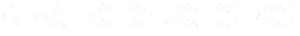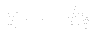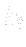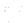Previous: Momentum equation Up: Balance equations in fluid Next: Equation of state

This is an automatically generated documentation by LaTeX2HTML utility. In case of any issue, please, contact us at info@cfdsupport.com.

## Energy equation

Energy equation is not necessarily needed for our purposes. But we mention it to be complete. This equation describes the conservation of internal energy in fluid.(21.11)

The heat flux is usually described by Fourier’s law:grad(21.12)

Hereis the thermal diffusion coefficient andis the absolute temperature. For incompressible flow, the energy equation is decoupling and can be solve separately when needed.